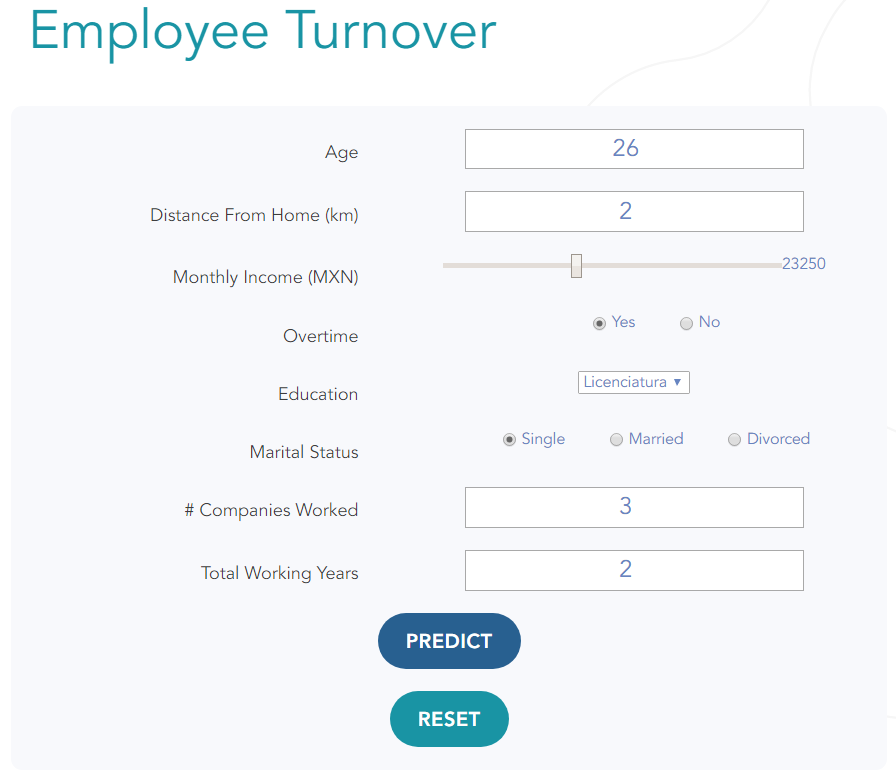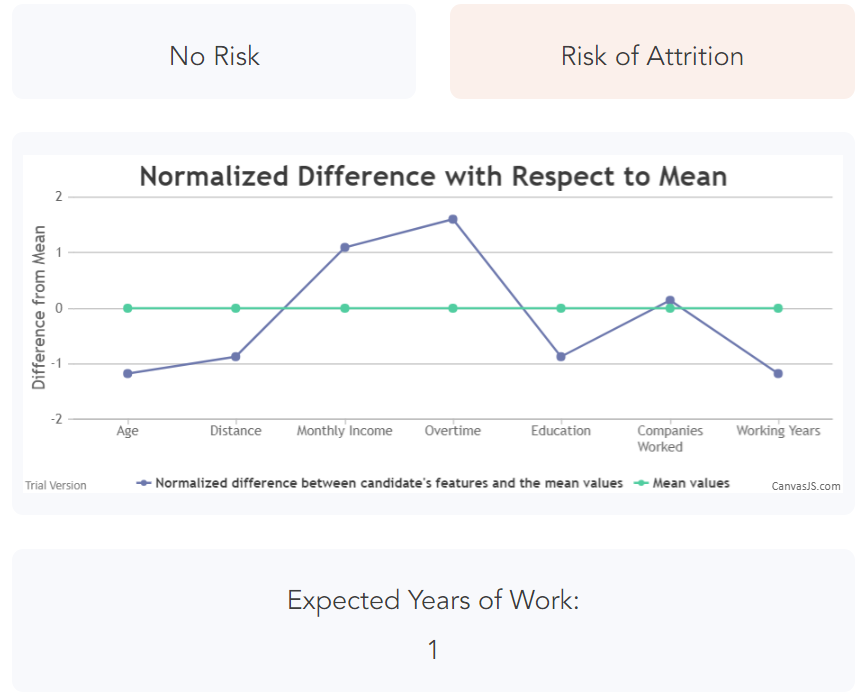{{announcement.body}}
{{announcement.title}}

# Employee Turnover Prediction With Deep Learning

DZone 's Guide to

# Employee Turnover Prediction With Deep Learning

### Learn about a neural network model that is capable of identifying employee candidates with a high risk of turnover, accomplishing this task with around 96% accuracy.

· AI Zone ·
Free Resource

Comment (8)

Save
{{ articles.views | formatCount}} Views

Mexico ranks eighth in the world for employee turnover, with an average turnover rate of about 17% each year — and some industries, like food service, getting up to 50%. According to a study from Catalyst, the cost of replacing an employee is around 50% to 75% of the employee’s annual salary, on average. Considering a mid-level position with a monthly salary of 20,000 pesos, the total cost of replacing this employee would be around 140,000 pesos. On average, it takes around 50 days to replace an employee, and the costs incurred due to productivity loss will keep adding up. For a big company like everis with over 20,000 employees, considering a turnover rate of 15% and an average salary of \$15,000 pesos, the total annual cost of turnover would rise up to at least 270 million pesos.

In this article, we provide details about a neural network model that is capable of identifying employee candidates with a high risk of turnover, accomplishing this task with around 96% accuracy.

## Methodology

We used the dataset HR Employee Attrition and Performance, a fictional dataset created by IBM data scientists. It contains 1,470 rows of employee historical data.

An exploratory data analysis was done to identify features that had the highest correlation with employee turnover. These are the most important features we found:

• Age
• Distance from home
• Overtime
• Education
• Marital status
• Number of companies worked at
• Total working years
• Monthly income

These features were used to train the model to predict turnover risk. The dataset already contained a feature called attrition, which indicated whether the employee left the position and had to be replaced. This feature was one-hot encoded (showed after splitting data into training and testing sets) and was used as the target for the neural network’s predictions. Here are the helper functions used for one-hot encoding:

``````from sklearn.preprocessing import StandardScaler, OneHotEncoder, LabelEncoder

### One Hot Encoding
def one_hot_values(values):
'''
takes array and returns one hot encoding with label encoder for inverse transform
'''
label_encoder = LabelEncoder()
integer_encoded = label_encoder.fit_transform(values)
onehot_encoder = OneHotEncoder(sparse=False)
integer_encoded = integer_encoded.reshape(len(integer_encoded), 1)
onehot_encoded = onehot_encoder.fit_transform(integer_encoded)
return onehot_encoded, label_encoder

def inverse_one_hot(label_encoder, one_hot):
inverse = []
for i in range(len(one_hot)):
inverse.append(label_encoder.inverse_transform([np.argmax(one_hot[i, :])]))
return inverse``````

Due to the unbalanced nature of the dataset (employees labeled with turnover represented around 16% of the population, or 237 of 1,470 cases), an upsample technique was used to repeat turnover cases — so the data had 1,233 cases with turnover and 1,233 cases without turnover.

Upsampling the dataset avoids a situation where the model learns to predict "no turnover" every time; in this case it would achieve around 84% of accuracy by doing so (this accuracy serves as our baseline).

``````# Separate majority and minority classes

# Upsample minority class
replace=True,     # sample with replacement
n_samples=len(data_empleados_majority),    # to match majority class
random_state=123) # reproducible results

# Combine majority class with upsampled minority class

# Display new class counts

Next, a `StandardScaler` was used to normalize data to ranges from -1 to 1 to avoid outliers to affect the predictions in a disproportionate way.

``````class standard_scaler:
def __init__(self, name):
self.name = name # candidato o empleado
self.scalers = {} # asignar cada scaler con el nombre de la columna (ej.'Age')
self.scalers[name] = scaler

# Initialize a standard_scaler class to hold all scalers for future reverse scaling

def scale_and_generate_scaler(data):
standard_scaler = StandardScaler()
scaled = standard_scaler.fit_transform(data.astype('int64').values.reshape(-1, 1))
return scaled, standard_scaler

def scale_array(scaler, array):
return scaler.transform([array])

def inverse_scale_array(scaler, array):
return scaler.inverse_transform([array])

def scale_append(data, scalers, name):
scaled, scaler = scale_and_generate_scaler(data[name])
return scaled, scalers

# Select features to scale
'Age',
'DistanceFromHome',
'EnvironmentSatisfaction',
'JobInvolvement',
'JobSatisfaction',
'MonthlyIncome',
'OverTime_Num',
'YearsAtCompany',
'WorkLifeBalance',
'Education',
'MaritalStatus_Num',
'NumCompaniesWorked',
'RelationshipSatisfaction',
'TotalWorkingYears'
]

# Scale each feature, save each feature's scaler

After having the data prepared, it was randomly split into training data (80%) and testing data (20%), using a random seed for reproducibility.

``````X_train, X_test = train_test_split(data_empleados_upsampled, test_size=0.2, random_state=RANDOM_SEED)

y_train = X_train['Attrition_Num']
X_train = X_train.drop(['Attrition_Num', 'Attrition', 'BusinessTravel', 'OverTime', 'MaritalStatus', 'YearsAtCompany'], axis=1)

y_test = X_test['Attrition_Num']
X_test = X_test.drop(['Attrition_Num', 'Attrition', 'BusinessTravel', 'OverTime', 'MaritalStatus','YearsAtCompany'], axis=1)

X_train = X_train.values
X_test = X_test.values

# One-Hot encoding
y_train_hot, label_encoder = one_hot_values(y_train)
y_test_hot, label_encoder_test = one_hot_values(y_test)``````

Then, the neural network was built using Keras. Its architecture is the following:

``````nb_epoch = 200
batch_size = 64

input_d = X_train.shape
output_d = y_train_hot.shape

model = Sequential()

sgd = SGD(lr=0.01, decay=1e-6, momentum=0.9, nesterov=True)
rms = 'rmsprop'
model.compile(loss='categorical_crossentropy',
optimizer=sgd,
metrics=['accuracy'])``````

It’s a simple three-layer neural network, with two neurons at the last layer to predict the correct class based on the one-hot encoded message used as the target for the model; no attrition = [0,1] and attrition = [0,1].

A stochastic gradient descent optimizer was used with a learning rate of 0.01, a batch size of 64, and a loss function of `categorical_crossentropy`. It was trained for 200 epochs, achieving a validation accuracy of 96.15% (compared to baseline 84% for always predict turnover).

The output for each prediction is an array of size 2; the elements of this array sum up to 1. For extracting the predicted class, the highest element from the array is taken. If the first element is larger, the predicted class has no attrition. Similarly, the predicted class has attrition if the second element is larger than the first. The function `inverse_one_hot()` is used to get the predicted class from one-hot encoded model predictions. Here's an example:

``````# Get predictions
preds = model.predict(X_test)

# Reverse the One-Hot encoding
res = [inverse_one_hot(label_encoder_test,np.array([list(preds[i])])) for i in range(len(preds))]``````

Here's an example of how to perform prediction on a custom profile; let's say, a potential candidate for a job:

``````perfil = ['26', '2', '1', '1', '1', '1', '2500', '1', '1', '2', '2', '6', '1', '2']

data_vars = [
'Age',
'DistanceFromHome',
'EnvironmentSatisfaction',
'JobInvolvement',
'JobSatisfaction',
'MonthlyIncome',
'OverTime_Num',
'WorkLifeBalance',
'Education',
'MaritalStatus_Num',
'NumCompaniesWorked',
'RelationshipSatisfaction',
'TotalWorkingYears']

scaled_input = []
# Note: the scalers_empleados object can be saved with pickle.dump for later use.
for i in range(len(perfil)):
scaled_input = np.array(scaled_input).flatten().reshape(1,len(data_vars))

pred = model.predict(scaled_input)
res = [inverse_one_hot(label_encoder,np.array([list(pred[i])])) for i in range(len(pred))]
res = np.reshape(res, len(res))
print({'pred':str(res)})``````

A similar neural network was built to predict `YearsAtCompany`, a variable that corresponds to the total number of years the employee is expected to last in the company. The architecture is the same, with the exception of the last layer, which has five neurons instead of two to predict 0, 1, 2, 4 or 10 years.

It was trained for 350 epochs and yielded an accuracy of 82.52% (compared to baseline 20% for a random guess, a 1/5 chance; or 22% for always predicting four years).

Here's a function to get insights into the model's performance:

``````def evaluate_model(model, X_train):
preds = model.predict(X_train)
y_train_inv = [inverse_one_hot(label_encoder_train,np.array([list(y_train_hot[i])])) for i in range(len(y_train_hot))]
y_train_inv = np.array(y_train_inv).flatten()
preds_inv = [inverse_one_hot(label_encoder_train,np.array([list(preds[i])])) for i in range(len(preds))]
preds_inv = np.array(preds_inv).flatten()

correct = 0
over = 0
under = 0
errors = []
for i in range(len(preds_inv)):
if preds_inv[i] == y_train_inv[i]:
correct += 1
elif (preds_inv[i]) < (y_train_inv[i]):
under += 1
elif (preds_inv[i]) > (y_train_inv[i]):
over += 1
errors.append(((preds_inv[i]) - (y_train_inv[i])))
print("correct: {}, over {}, under {}, accuracy {}, mse {}".format(correct, over, under, round(correct/len(preds_inv),3), np.round(np.array(np.power(errors,2)).mean(),3)))
print("errors:",pd.Series(np.array([abs(i) for i in errors if i != 0])).describe())
print("preds",pd.Series(preds_inv).describe())
print("y_train",pd.Series(y_train_inv).describe())
print(pd.Series(preds_inv).value_counts())
print(pd.Series(y_train_inv).value_counts())
print(sns.boxplot(np.array([abs(i) for i in errors if i != 0])))

return y_train_inv, preds_inv``````

## Implementation and Results

A front-end was designed for the user to input characteristics of a candidate, and both models will yield a result: first identifying if the candidate has a risk of turnover and then predicting how many years the candidate would be predicted to stay at the potential position..A graph is also created to compare the normalized values of the candidates' characteristics with the average value of all the employees in the dataset. This is used to see which characteristics differ the most from the normal values.## Conclusion

There is still work to be done. It would be great to test these models with real data coming from a Mexican company. Also, the features that have a higher correlation with turnover risk may differ from dataset to dataset. Additionally, other features could be added in, like monthly grocery and housing expenses, as well as a more detailed analysis based on industry and the position for which the candidate applies.

The expected years at company prediction could also be a little more specific, especially for the first two years. Right now, the model can only predict one year or another, but maybe it would be worthwhile to predict months instead of years to differentiate candidates using more information.

Still, recruiters could greatly benefit from these kinds of tools; they can have objective information at hand to make more informed decisions, and if the candidate turns out to have a high risk of turnover, at least it’s something that can be discussed directly with the candidate to negotiate how both parties can benefit. With these tools and novel strategies to combat turnover, companies around the world could reduce their turnover significantly, potentially increasing their income by millions.

Topics:
deep learning ,ai ,tutorial ,machine learning ,neural network ,predictive analytics

Comment (8)

Save
{{ articles.views | formatCount}} Views

Opinions expressed by DZone contributors are their own.